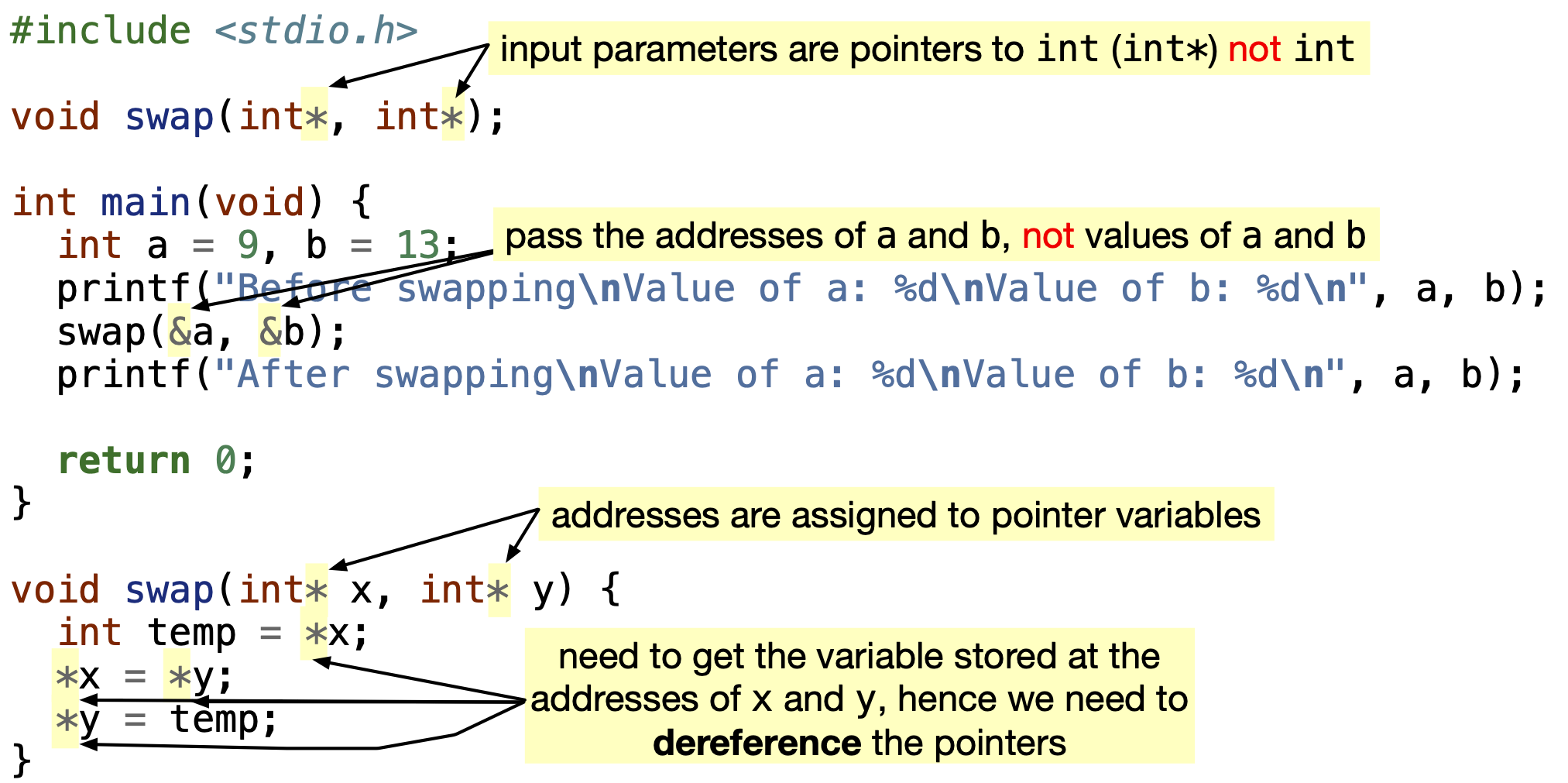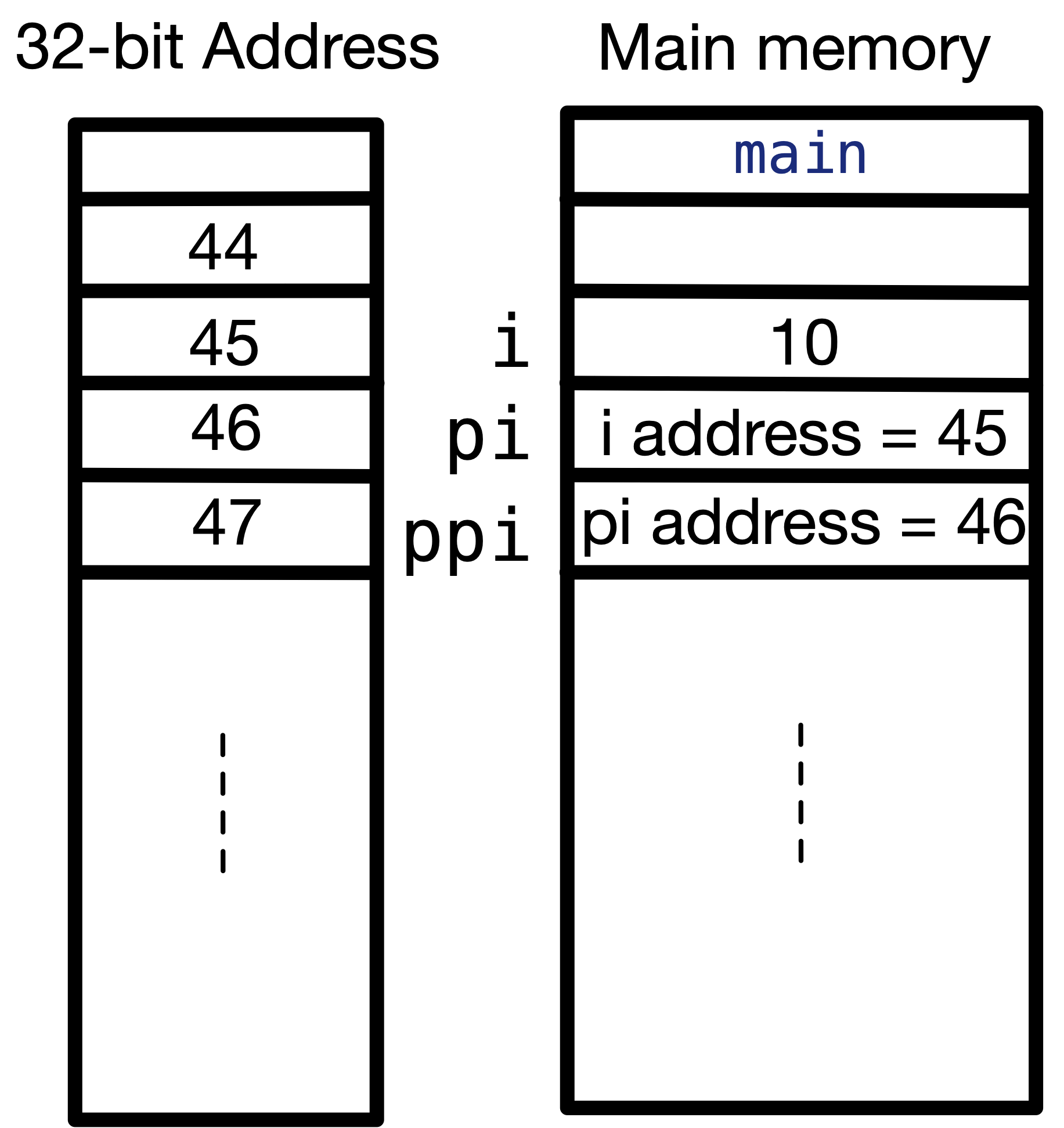# 6.3. How to use pointers to communicate more with functions?#

Now that we know what are pointers and how to use them, we will use pointers to solve the issue with swapping two variables in a function. In the following figure, we highlight the additions we made to the source code where we called swap function by value (not by pointers). Source code can be downloaded from here: swapping.c, and can be copied from below.Fig. 6.5 Highlight additions in code when we changed the swap function to call it by pointers, not by value.#

Code

 1#include <stdio.h>
2
3void swap(int*, int*);
4
5int main(void) {
6  int a = 9, b = 13;
7  printf("Before swapping\nValue of a: %d\nValue of b: %d\n", a, b);
8  swap(&a, &b);
9  printf("After swapping\nValue of a: %d\nValue of b: %d\n", a, b);
10
11  return 0;
12}
13
14void swap(int* x, int* y) {
15  int temp = *x;
16  *x = *y;
17  *y = temp;
18}


Output

Before swapping
Value of a: 9
Value of b: 13
After swapping
Value of a: 13
Value of b: 9


In the following video, we trace the program to see how it works.

In line $$3$$, we changed the prototype to pass pointers as input parameters. The pointers data types are written as int*.

In line $$8$$, instead of swap(a, b);, we are passing the addresses of a and b using swap(&a, &b);, because swap function now takes pointers that stores addresses, not int values.

In lines $$14$$ to $$17$$, we are dealing with the values stored in the addresses of x and y, hence we have to dereference x and y. *x accesses the value of a in the main function memory space in the main memory. Similarly, *y accesses the value of b stored in the main function memory space.

## 6.3.1. What is the size of the pointer variable?#

We discussed before that old machines represent addresses using 32 bits, while modern machines use 64 bits. Let’s test what does my computer uses. I tried running the following code, where I print the size of pointers to main data types we use such as int*, double*, char* and bool*. All pointers require 8 bytes to store the values of addresses, i.e. 64 bits. Try out the code by downloading size-of-pointer.c, and can be copied from below.

Code

#include <stdbool.h>
#include <stdio.h>

int main(void) {
printf("Size of pointer (int*) is %d.\n", sizeof(int *));
printf("Size of pointer (double*) is %d.\n", sizeof(double *));
printf("Size of pointer (bool*) is %d.\n", sizeof(bool *));
printf("Size of pointer (char*) is %d.\n", sizeof(char *));
return 0;
}


Output

Size of pointer (int*) is 8.
Size of pointer (double*) is 8.
Size of pointer (bool*) is 8.
Size of pointer (char*) is 8.


## 6.3.2. Can a pointer hold the address of another pointer?#

A pointer can hold the address of another pointer, since they are all addresses. However, if we have a pointer that holds address of an int its type is int*, and the type of the pointer that holds the address of that int* pointer is int** – an additional * is added. For example,

Code

#include <stdio.h>

int main(void) {
int i = 0;
int *pi;
int **ppi;

pi = &i;
ppi = &pi;
i = 10;

printf("What i is storing: %d\n", i);
printf("What pi is pointing to: %d\n", *pi);
printf("What (ppi is pointing to) = pi is pointing to: %d\n", **ppi);
return 0;
}


Output

What i is storing: 10
What pi is pointing to: 10
What (ppi is pointing to) = pi is pointing to: 10


In the main memory, the values of i, pi and ppi are shown in the following figure. Please note that addresses are arbitrary addresses.Fig. 6.6 How does a pointer to a pointer look like in the memory? It just holds another address.#

Important!

It is important to note that int* pi; is equivalent to int *pi;, and

int *pi = &i;


is equivalent to

int *pi;
pi = &i;


## 6.3.3. Can a function return a pointer?#

Let’s write a function that returns a pointer to the variable with the maximum value. The function takes in two pointers to two double variables and returns one pointer to the largest double.

Code

 1#include <stdio.h>
2double* largestValLoc(double*, double*);
3
4double* largestValLoc(double* a, double* b) {
5  double* temp;
6  if (*a > *b) {
7    temp = a;  // temp is double* and a is double*, so temp = a is permissible
8  } else {
9    temp = b;
10  }
11  return temp;  // temp is double*, and return type is double*
12}
13
14int main(void) {
15  double x = 2.6, y = 7.3;
16  double* p =
17      largestValLoc(&x, &y);  // pass address of x to a, and address of y to b
18  // p is (double*) and largestValLoc returns (double*)
19  printf("Address of x: %p having value %.1lf.\n", &x, x);
20  printf("Address of y: %p having value %.1lf.\n", &y, y);
21  printf("Address of larger variable: %p.\n", p);
22  return 0;
23}


Output

Address of x: 0x304757170 having value 2.6.
Address of y: 0x304757168 having value 7.3.


## 6.3.4. Initialization Vs. Declaration of a pointer variable#

As we discussed earlier in Declaring Vs. Initializing Variables, if you declare a variable without initializing it as in int var;, it is unknown what is the value of var. var holds a “garbage” value. Similarly, with pointers, if you declare a pointer without initializing it, it holds a “garbage” address.

For example, in the following program, we try using an uninitialized pointer. The warning message printed is “warning: variable ‘p’ is uninitialized when used here” as shown in the figure.

Code

1#include <stdio.h>
2
3int main() {
4  int* p;
5  *p = 5;
6
7  return 0;
8}


Why is this an issue? The problem is if you ignore this error, and try to dereference p as in line 5 *p = 5;, you will be dereferencing a garbage address that may (or may not) exist. The behavior of your program is undefined.

1. If the address exists, but your program can not access or does not have permission to access, the operating system will raise an error named “Segmentation Fault” error.

2. If the address exists, and your program can access it (rarely happens), it may change a value of another variable in your program.

3. If the address does not exist, your program may fail silently. This means it may not print statements in a printf, or it may not take input from the user in a scanf.

In short, do not use an uninitialized pointer. This is why the compiler recommends you set the address of the pointer to NULL when you declare it.

What is NULL? NULL is defined pointer constant, which corresponds to $$0$$. In other words, NULL pointer is not pointing to any valid address.

What happens if you dereference a NULL pointer? The behavior of your program is undefined.

Then what is the benefit of initializing my pointer to NULL? The benefit of initializing a pointer to NULL is that before you dereference it, you can check if it is NULL or not. If it is NULL, then it does not have a valid address, and you shouldn’t dereference it. Otherwise, you can dereference it. For example, the following program checks if a pointer is NULL or not before dereferencing it. This good practice of checking if a pointer is NULL or not will be handy when we discuss “Linked Lists”.

Code

#include <stdio.h>

int main() {
int* p = NULL;

if (p == NULL) {
printf("Cannot dereference it!\n");
} else {
printf("The value at address p is %d.\n", *p);
}

return 0;
}


Output

Cannot dereference it!


## 6.3.5. Practice Problem solved Winter 2018 Midterm Exam Q7#

What is the output of the following program? The solution is discussed in the video above.

Code

#include <stdio.h>
int *confuse(int *x, int *y) {
(*y)++;
y = x;
*y = 10;
return (y);
}

int main(void) {
int a = 6, b = 7;
int *f = &b;
f = confuse(&a, &b);
(*f)++;
printf("a = %d and b = %d\n", a, b);
return 0;
}


Output

a = 11 and b = 8